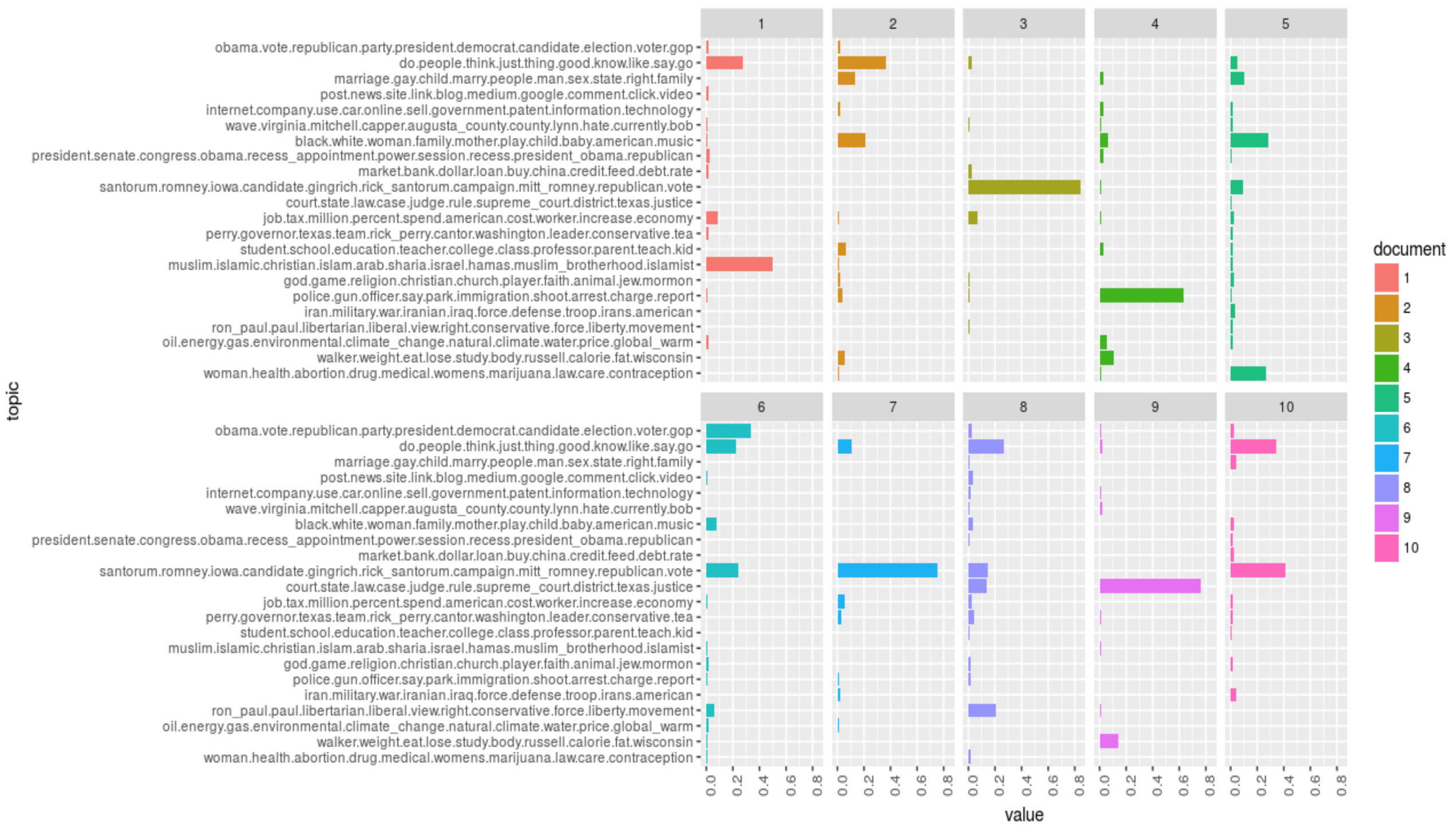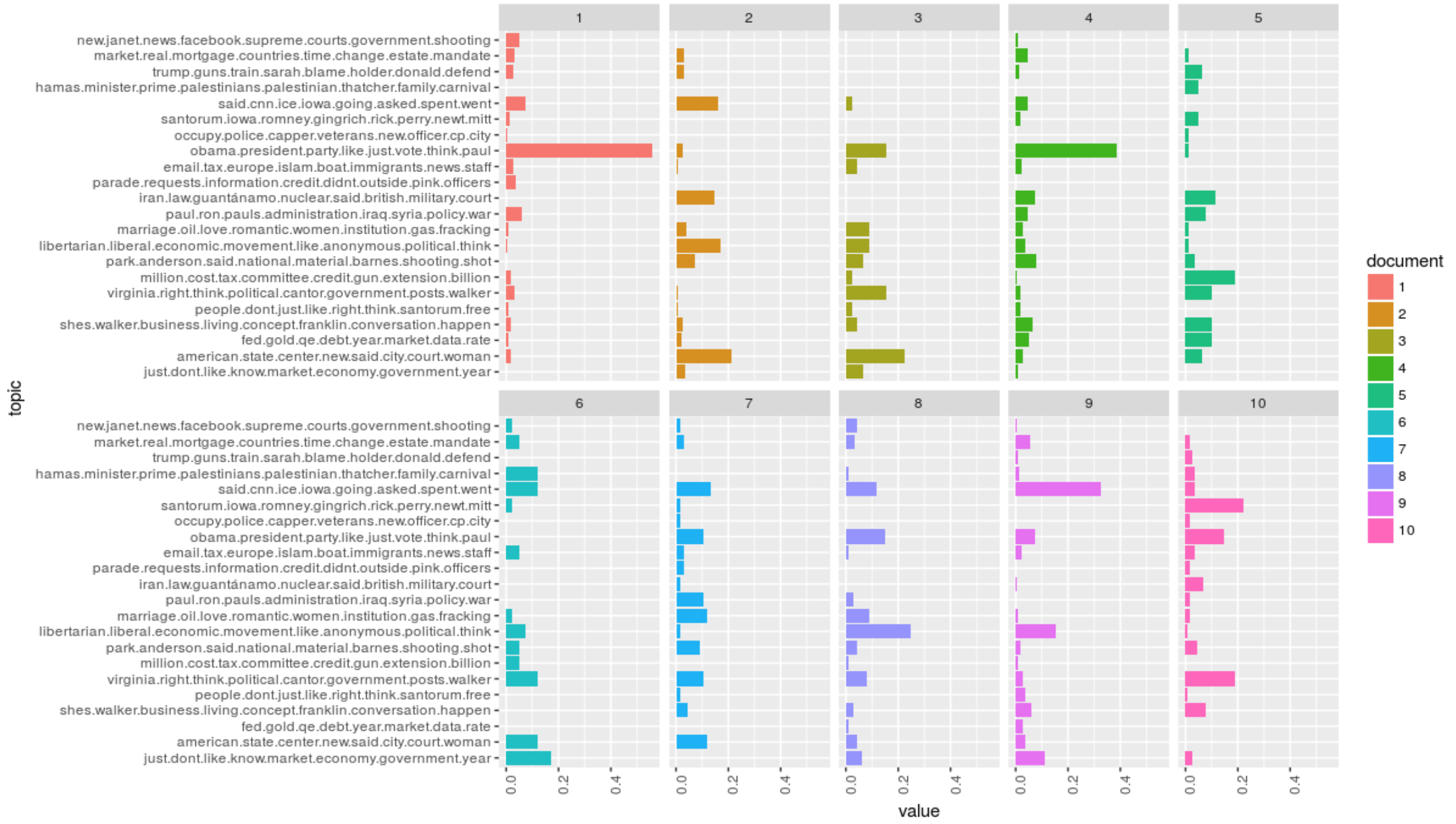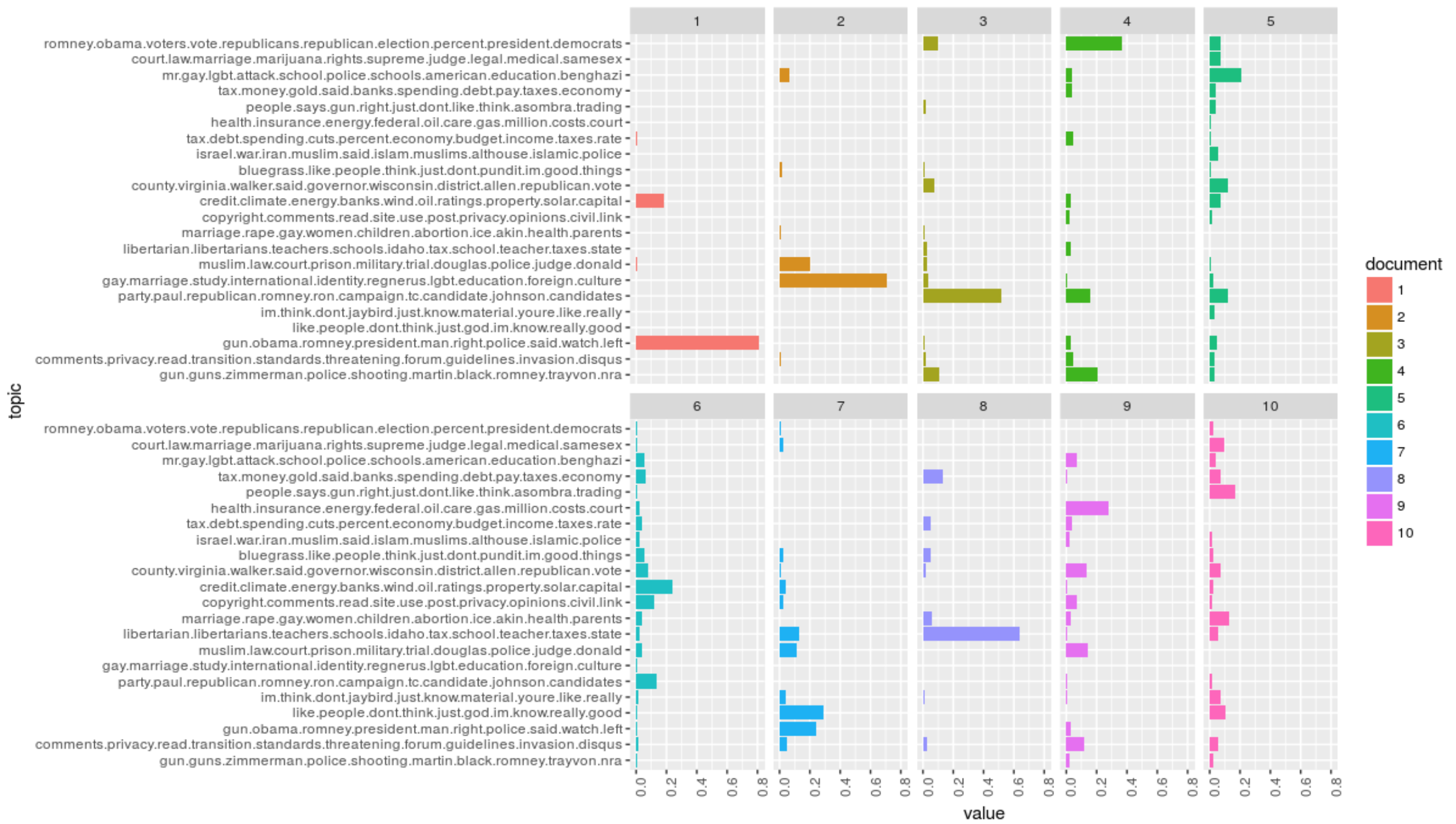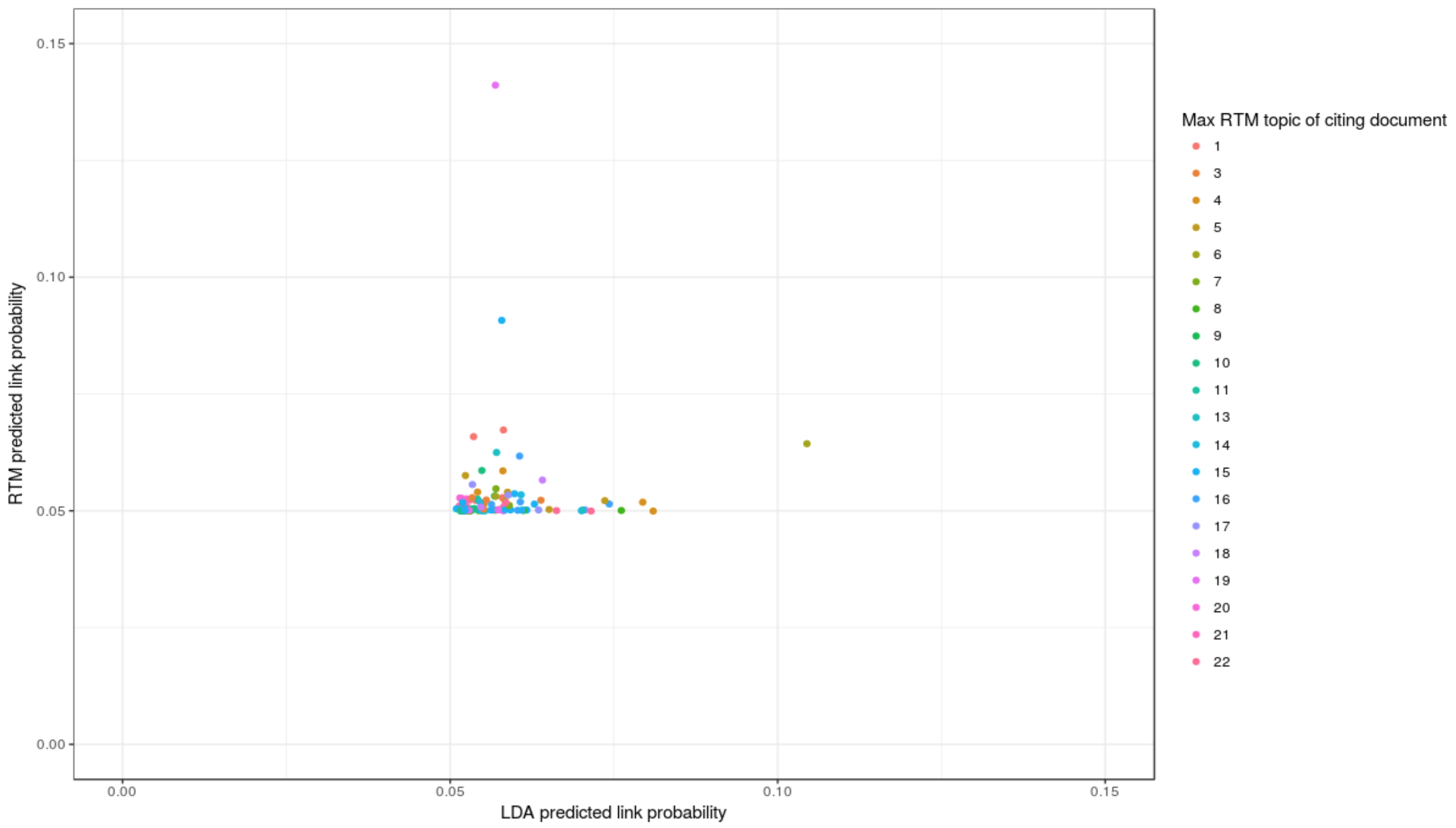Use different Topic Modeling approaches on Political Blogs to see the performance of diverse methods.

# Introduction

## Key Values in Cleaned Blog Posts

After preprocessing the text extracted from blog posts:

• dates: string of the given date in mm/dd/yy format
• domains: string of the blog website where post was found (remove “www.”)
• links: string of other websites occured in the post as hyperlinks (sorted alphabetically)
• words: filtered words from raw text in the blog posts (TFIDF variance threading used)
• rawText: direct content from blog posts (remove short posts and duplicate posts )
• words_stem: stemmed words using Hunspell stemmer (e.g., apples -> apple)

# Analysis via several Topic Modeling Methods

## General LDA

General LDA Model via Collapsed Gibbs Sampling Methods for Topic Models:## Supervised LDA

Here use Blog Site as labels.## Relational Topic Model

RTM models the link as binary random variable that is conditioned on their text. The model can predict links between documents and predict words within them. The algorithm is based on variational EM algorithm.

1. For each document $d$:
1. Draw topic proportions $\theta_d|\alpha \sim \text{Dir}(\alpha)$
2. For each word $w_{d,n}$:
• Draw assignment $z_{d,n}|\theta_d \sim \text{Mult}(\theta_d)$
• Draw word wd,n | zd,n, $\beta$1:K$\sim \text{Mult}(\beta$zd,n$)$
2. For each pair of documents $d,d’$:
• Draw binary link indicator $y|z_d,z$ d’ $\sim \psi (\cdot | z_d,z$ d’ $)$Compare the performance of link prediction with the one of LDA. The plot below shows the predicted link probabilities from RTM against the ones of LDA for each document, and also shows the most expressed topics by the cited document. (sample 100)